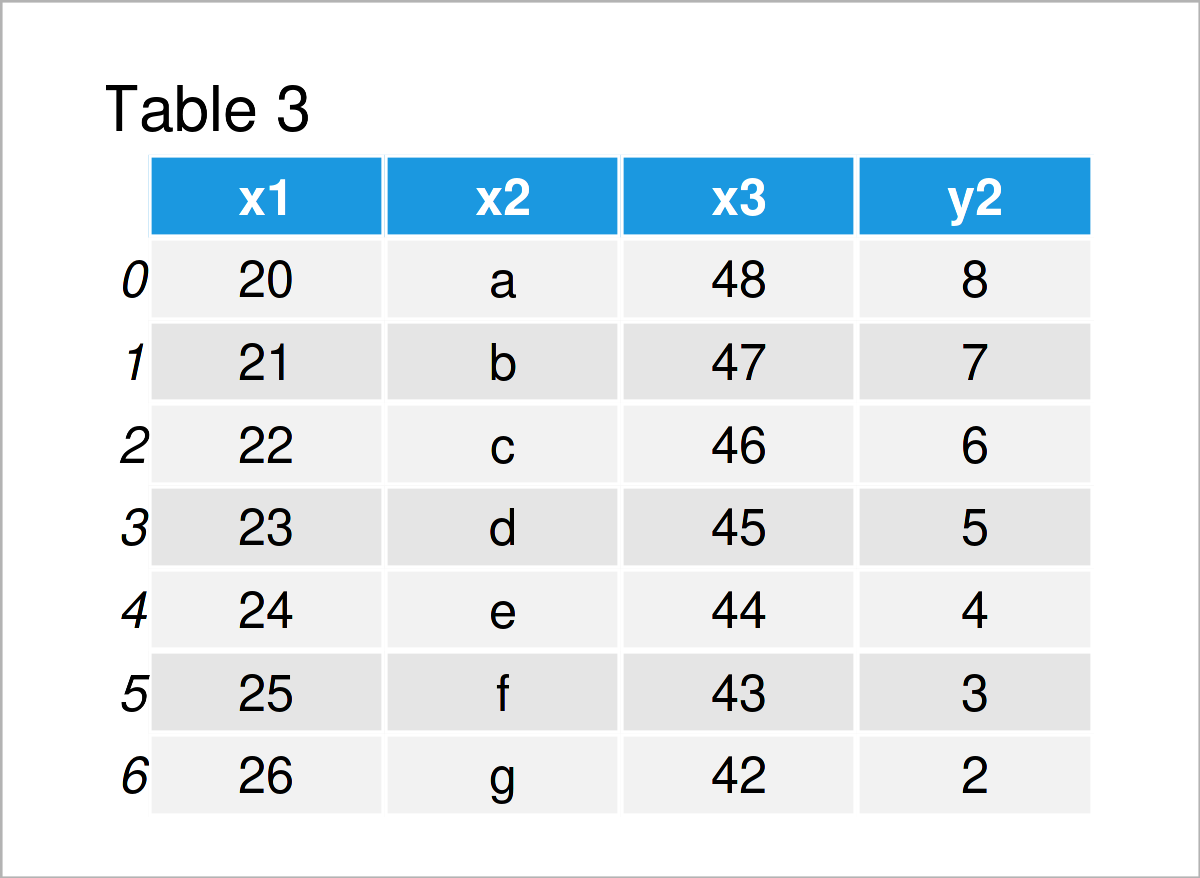# Add Column from Another pandas DataFrame in Python (Example)

In this Python programming tutorial you’ll learn how to append a column from another pandas DataFrame.

Let’s start right away!

## Example Data & Add-On Libraries

First, we have to import the pandas library to Python:

`import pandas as pd # Import pandas library`

The following data is used as basement for this Python tutorial:

```data1 = pd.DataFrame({"x1":range(20, 27), # Create first pandas DataFrame "x2":["a", "b", "c", "d", "e", "f", "g"], "x3":range(48, 41, - 1)}) print(data1) # Print first pandas DataFrame``````data2 = pd.DataFrame({"y1":range(1, 8), # Create second pandas DataFrame "y2":range(8, 1, - 1)}) print(data2) # Print second pandas DataFrame```As shown in Tables 1 and 2, the previous Python programming code has created two pandas DataFrames with different columns. Both of these data sets have the same number of rows.

## Example: Append Column from Another pandas DataFrame

This example shows how to add a variable from another pandas DataFrame as a new column to a DataFrame in Python.

For this task, we can use the Python code below:

```data_new = data1.copy() # Create copy of first DataFrame data_new["y2"] = data2["y2"] # Add column from second to first print(data_new) # Print updated DataFrame```After running the previous syntax the new pandas DataFrame called data_new you can see in Table 3 has been created. It contains the values of the first input data set as well as the variable y2 of the second data set.

## Video, Further Resources & Summary

In case you need more explanations on how to union a column from another data set with an already existing pandas DataFrame, I recommend watching the following video on my YouTube channel. In the video, I’m explaining how to combine a column and a pandas DataFrame using the Python code of this page in the Python programming language:

Please accept YouTube cookies to play this video. By accepting you will be accessing content from YouTube, a service provided by an external third party.If you accept this notice, your choice will be saved and the page will refresh.

In addition, you may want to have a look at some of the other articles on my website. I have released several related articles already.

In this article, I have explained how to join and add a column from another pandas DataFrame in Python. In case you have additional questions on how to merge a new variable to a pandas DataFrame, tell me about it in the comments. Furthermore, please subscribe to my email newsletter in order to get updates on new tutorials.

Subscribe to the Statistics Globe Newsletter